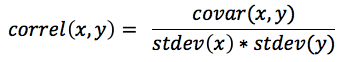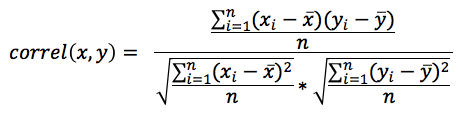# CORREL

Correlation indicates the degree of association between two sets of values. The CORREL function returns correlation coefficient that varies between -1 and 1.

• A value of 1 indicates that as X increases, Y always increases.
• A value of -1 indicates that as X increases, Y always decreases.
• A value of 0 indicates that there is no correlation; values for X cannot be used as predictors for values of Y.

Correlation coefficient is measured by calculating the covariance of the two sets of values, which measures how they vary with each other. This measure is then divided by the product of the standard deviations of each set of values. See Covariance Functions and Standard Deviation Functions.

Correlation coefficient is a component calculation of the Least-Squares method of linear regression. See Least-Squares Functions.

CORREL function has the form `CORREL (numeric1,numeric2)` where numeric1 and numeric2 are facts or metrics.When this equation is broken down into its component parts, it becomes the following:Syntax

``````SELECT CORREL(…)
SELECT CORREL(metric1, metric2)
SELECT CORREL(metric1, fact2)
SELECT CORREL(fact1, fact2)
``````

Examples

``````SELECT CORREL(Production Volume, Rate of Defects)
SELECT CORREL(Gross Tonnage, Gross Profit)
``````